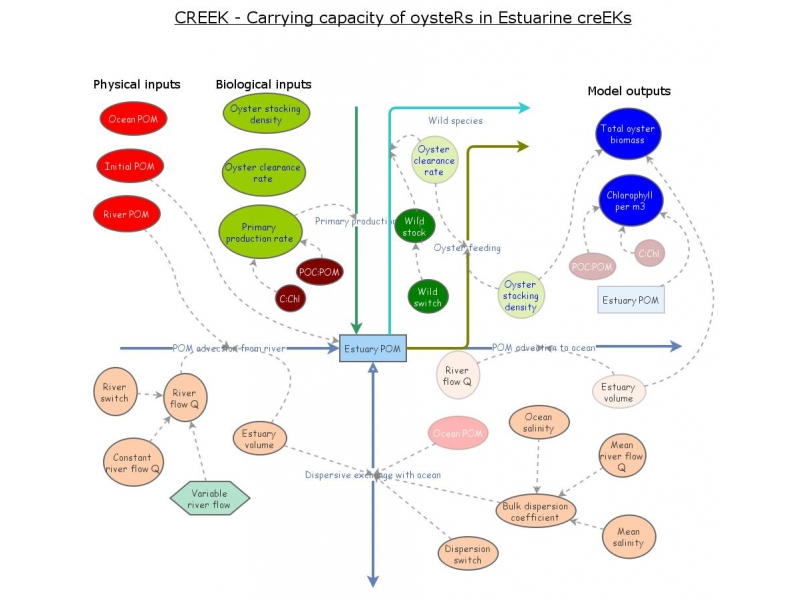CREEK - Carrying Capacity of OystersThis model implements a very simple shellfish carrying capacity simulation for tidal creeks with freshwater input.

Physics

The model implements the one-dimensional version of the advection-dispersion equation for an estuary. The equation is:

dS/dt = (1/A)d(QS)/dx - (1/A)d(EA)/dx(dS/dx) (Eq. 1)

Where S: salinity (or any other constituent such as chlorophyll or dissolved oxygen), (e.g. kg m-3); t: time (s); A: cross-sectional area (m2); Q: river flow (m3 s-1); x: length of box (m); E: dispersion coefficient (m2 s-1).

For a given length delta x, Adx = V, the box volume. For a set value of Q, the equation becomes:

VdS/dt = QdS - (d(EA)/dx) dS (Eq. 2)

EA/x, i.e. (m2 X m2) / (m s) = E(b), the bulk dispersion coefficient, units in m3 s-1, i.e. a flow, equivalent to Q

At steady state, dS/dt = 0, therefore we can rewrite Eq. 2 for one estuarine box as:

Q(Sr-Se)=E(b)r,e(Sr-Se)-E(b)e,s(Se-Ss) (Eq. 3)

Where Sr: river salinity (=0), Se: mean estuary salinity; Ss: mean ocean salinity

E(b)r,e: dispersion coefficient between river and estuary, and E(b)e,s: dispersion coefficient between the estuary and ocean.

By definition the value of E(b)r,e is zero, otherwise we are not at the head (upstream limit of salt intrusion) of the estuary. Likewise Sr is zero, otherwise we're not in the river. Therefore:

QSe=E(b)e,s(Se-Ss) (Eq. 4)

E(b)e,s = QSe/(Se-Ss) (Eq 5)

The longitudinal dispersion simulates the turbulent mixiing of water in the estuary during flood and ebb, which supplies salt water to the estuary on the flood tide, and make the sea a little more brackish on the ebb.

You can use the top slider to turn off dispersion (set to zero). If the variable being simulated is (a) salinity, you will see that if the tidal wave did not mix with the estuary water due to turbulence, the estuary would quickly become a freshwater system; (b) POM, then the ocean (which typically has less POM) will not contribute a flushing effect and the concentration of POM in the tidal creek or estuary will be higher.

The second slider allows you to simulate a variable river flow, and understand how dispersion compensates for changes in freshwater input.

Biology

Two biological functions are implemented in CREEK, both extremely simplified.

1. Primary production - a constant primary production rate is considered in gC m-3 d-1

2. Oyster filtration - a constant clearance rate (CR) is considered in L ind- 1 h-1, scaled to a certain stocking density S (ind m-3)

Units are normalized, and food depletion is CR * S * POM, in g POM m-3 d-1

The third slider allows for adjustment of different aquaculture densities.

Wild filter-feeding species are included in the model, using an identical clearance rate to the cultivated oysters. Wild species can be turned on or off in the model using the fourth slider.

The model provides three outputs:
1. POM concentration in mg L-1
2. Equivalent in chlorophyll (ug L-1)
3. Total oyster biomass in kg for the whole system